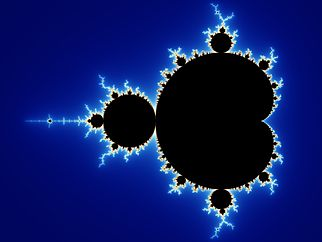Generic filters

## “The Mandelbrot Set” Benoit MandelbrotThe Mandelbrot Set by Benoit Mandelbrot is a fractal that employs the simple equation “z = z *z + c”.  This equation basically controls a feedback loop.  “The equation is calculated dozens, or even millions of times for each pixel. Each time through the loop, the result of one calculation is used as the input for the next calculation. It is this feedback loop that gives the Mandelbrot set, and many other fractals, their complex behaviour.”   The shape created occurs an infinite number of times and is rotated, distorted, and shrunken.  All the color bands used are connected throughout the entire piece and do not cross any other color bands as well.  The Mandelbrot set also requires millions of calculations and without a computer, it would be invisible even in the lowest of resolutions.  It also possesses infinte detail.  The viewer can zoom in as far as they want and the equation will still keep creating the shape and colors. 

This equation and many like it are now used to create a world of different fractals for man uses from art all the way up to musical visualization.